# Determine the force in members EL and LM of the truss and state if the members...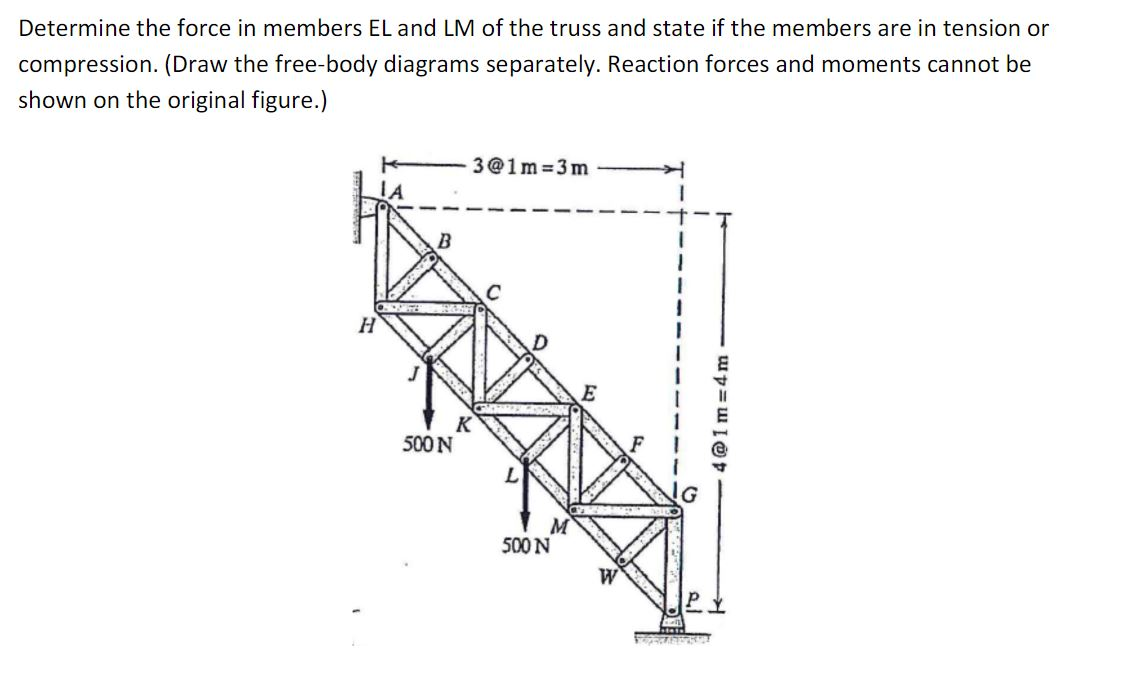Determine the force in members EL and LM of the truss and state if the members are in tension or compression. (Draw the free-body diagrams separately. Reaction forces and moments cannot be shown on the original figure.) [email protected] =3m 1 Н E [email protected]=4m 500N M 500N W OST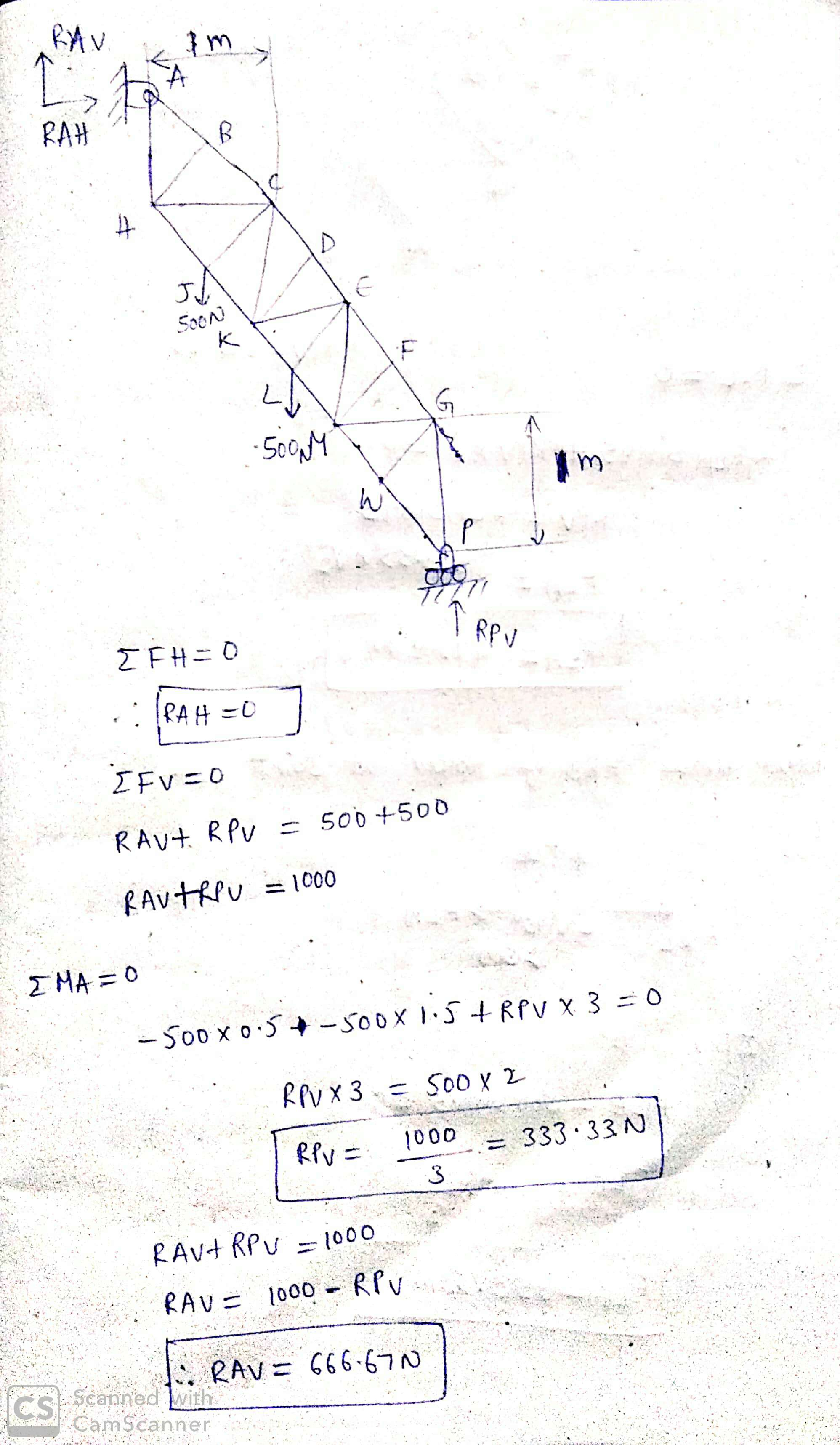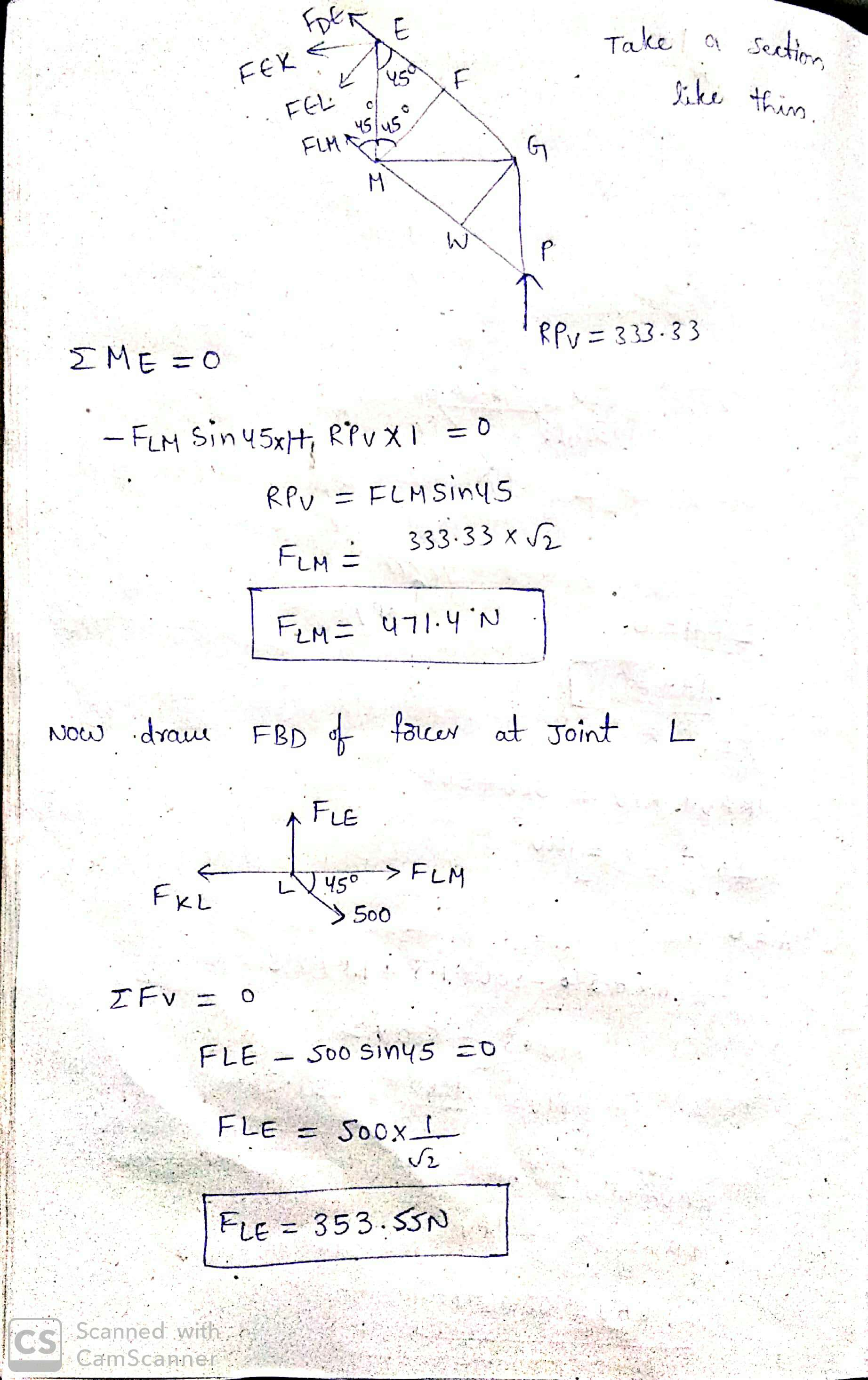##### Add Answer of: Determine the force in members EL and LM of the truss and state if the members...
Similar Homework Help Questions
• ### Determine the force in members EL and LM of the truss and state if the members...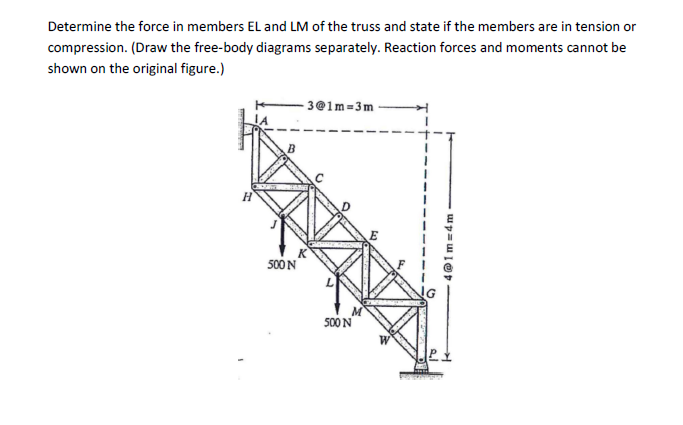Determine the force in members EL and LM of the truss and state if the members are in tension or compression. (Draw the free-body diagrams separately. Reaction forces and moments cannot be shown on the original figure.) [email protected] = 3m 41 m = 4 m 500 N 500N

• ### Determine the force in members EL and LM of the truss and state if the members...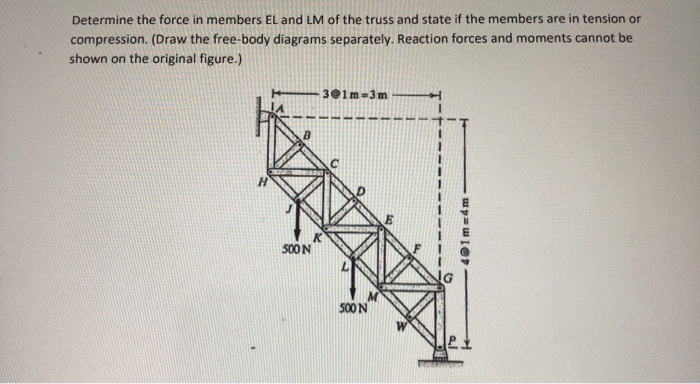Determine the force in members EL and LM of the truss and state if the members are in tension or compression. (Draw the free-body diagrams separately. Reaction forces and moments cannot be shown on the original figure.) 301m 3m 41 mm 500N 500N

• ### Determine the force in members EL and LM of the truss and state if the members...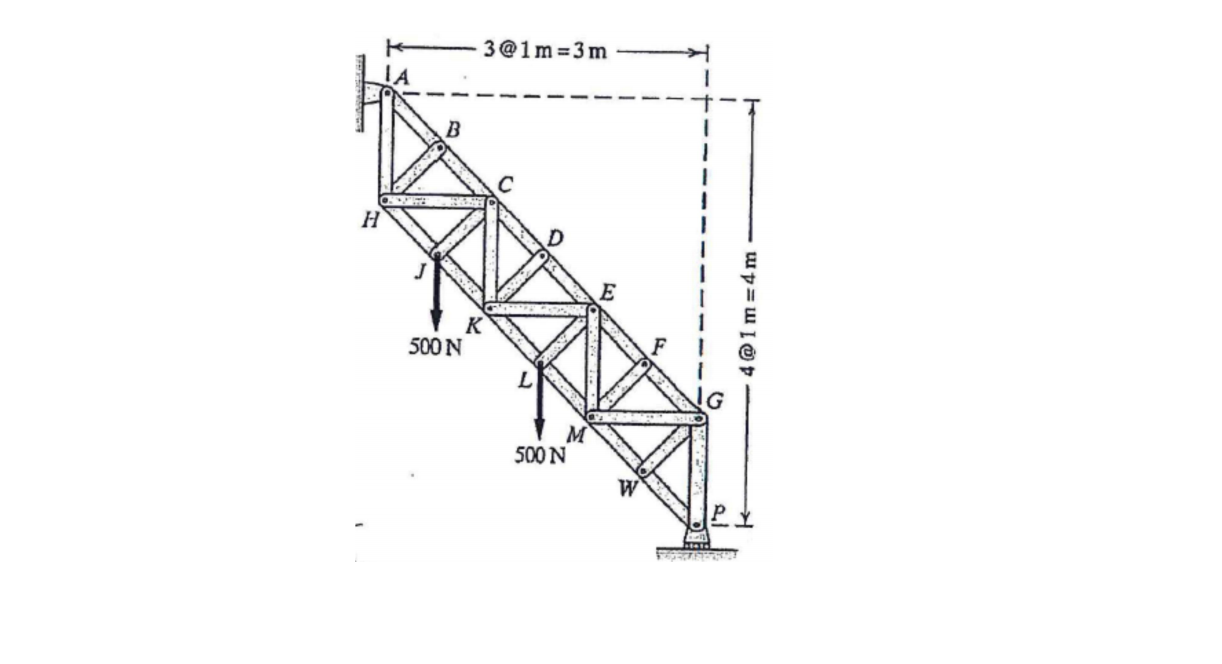Determine the force in members EL and LM of the truss and state if the members are in tension or compression. Draw FBD reaction snd moment forces [email protected] B Н [email protected] m=4m к 500N M 500 N W

• ### 1. Determine the forces in members DF and FE of the double-pitch roof truss shown. State...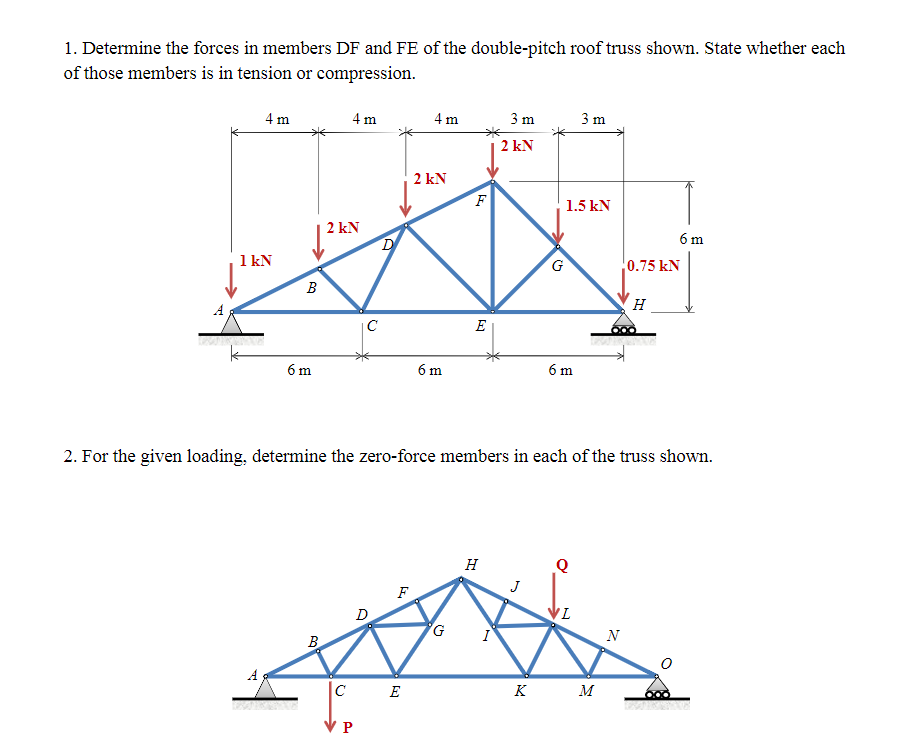1. Determine the forces in members DF and FE of the double-pitch roof truss shown. State whether each of those members is in tension or compression. 2. For the given loading, determine the zero-force members in each of the truss shown. 1. Determine the forces in members DF and FE of the double-pitch roof truss shown. State whether each of those members is in tension or compression. - 4m 4m 4m 3m 3m KV 6 m 0.75 kN 6m 6...

• ### Determine the force in each member of the truss and state if the members are in tension or compre...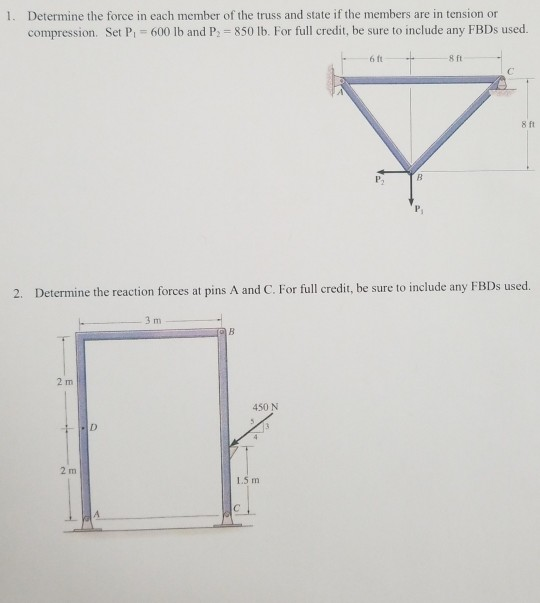Determine the force in each member of the truss and state if the members are in tension or compression. Set Pi # 600 lb and P-850 lb. For full credit, be sure to include any FBDs used. 1. 6 ft 8 ft 8 ft 2. Determine the reaction forces at pins A and C. For full credit, be sure to include any FBDs used. 3 m 2 m 450 N 1.5 m Determine the force in each member of the...

• ### Determine the force in members CD of the truss, and state if the member is in...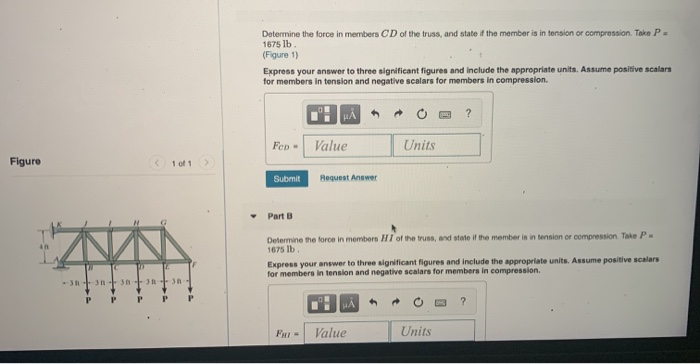Determine the force in members CD of the truss, and state if the member is in tension or compression. Take P. 1675 lb (Figure 1) Express your answer to three significant figures and include the appropriate units. Assume positive scalars for members in tension and negative scalare for members in compression. .: A O ? Feo - Value Units Figure 1 of 1 > Submit Request Answer Part 8 Determine the force in members of the true and state the...

• ### b) Determine the zero-force members. (1 mark) c) Determine the forces present in members IJ, IE...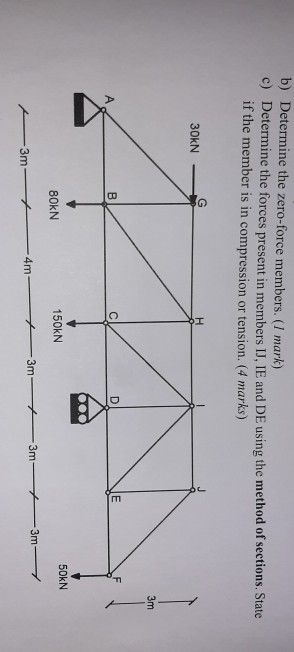b) Determine the zero-force members. (1 mark) c) Determine the forces present in members IJ, IE and DE using the method of sections. State if the member is in compression or tension. (4 marks) 30KN 80KN 50KN 150KN 3m- - 4m 4- 3m 3m 3m

• ### Question Four: For the pin-jointed truss shown in Figure 4, 3m 12 KN 3m 30 kN 3m 15 kN 4m 4m 3m 3m 6m Figure 4. Calcula...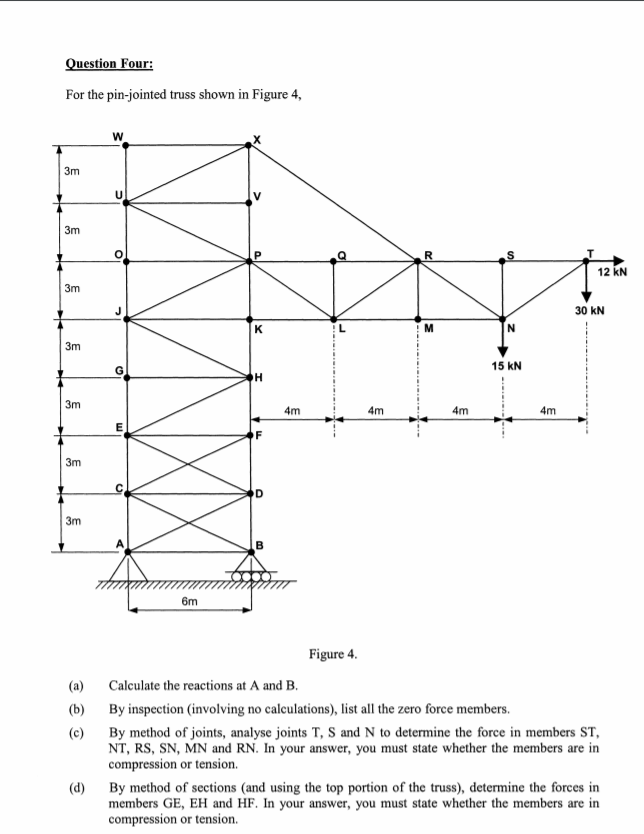Question Four: For the pin-jointed truss shown in Figure 4, 3m 12 KN 3m 30 kN 3m 15 kN 4m 4m 3m 3m 6m Figure 4. Calculate the reactions at A and B. (a) By inspection (involving no calculations), list all the zero force members. (b) (c) By method of joints, analyse joints T, S and N to determine the force in members ST NT, RS, SN, MN and RN. In your answer, you must state whether the members are...

• ### Problem 1 Determine the force in each member of the truss and state if the members...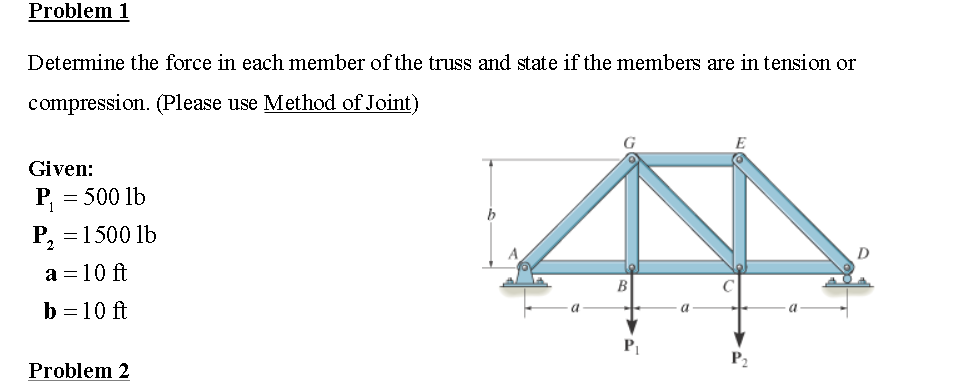Problem 1 Determine the force in each member of the truss and state if the members are in tension or compression. (Please use Method of Joint) Given: P, = 500 lb P, = 1500 lb a=10 ft b=10 ft Problem 2 Problem 3 Determine the force in members CD, CF, and GF of the truss. State if these members are in tension or compression. (Please use Method of Section) 5 kN 4 kN 4 kN 3 kN 2 kN (...

• ### Using method of joints, determine the force in each member of the truss and state if...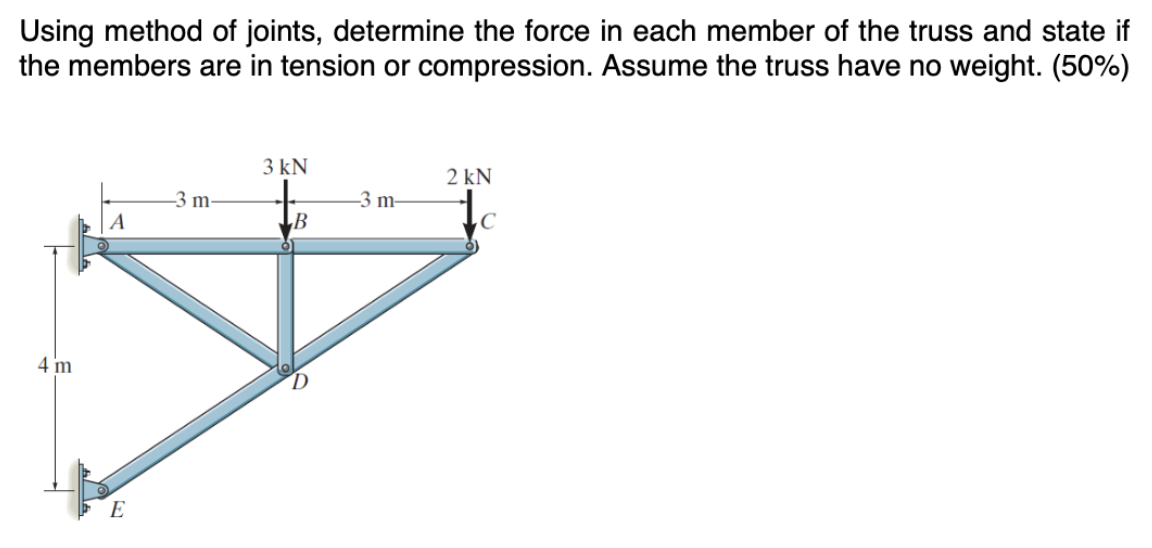Using method of joints, determine the force in each member of the truss and state if the members are in tension or compression. Assume the truss have no weight. (50%) 3 kN —3m— 3n 2 KN 1 12 m 4 m 0)

Free Homework App